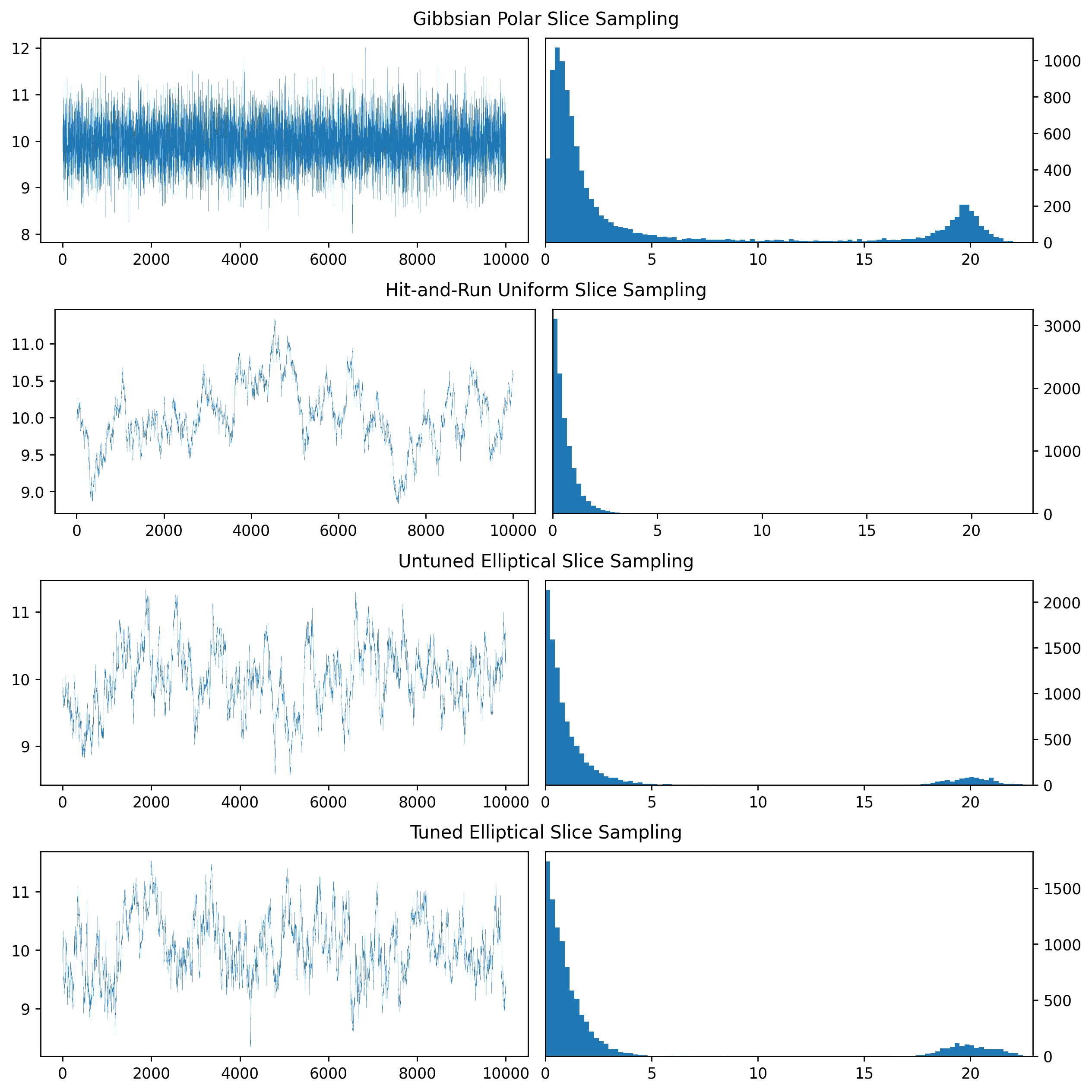# Hyperplane Disk Density $f_{\nu}(x) = \exp(-||x||^2 - (\sum_{i=1}^d x_i)^2)$¶

Density that is concentrated around a zero-centered disk of a hyperplane, i.e. a (d-1)-dimensional subspace.

In :
import sys
try:
if "pyodide" in sys.modules:
import piplite
await piplite.install('fastprogress')
except:

In :
import scipy
import slice_sampling as ss
import mcmc_utils as mcu
import plotting_functions as pfs
import numpy as np
import numpy.linalg as alg
import pandas as pd

In :
# Set parameters
d = 200 # dimension, should be > 1
itnum = int(1e4) # number of chain iterations
path_prefix = "./plots/hyperplane_disk_"

In :
tde_cnt = 0 # target density evaluation count

In :
def log_density(x):
global tde_cnt
tde_cnt += 1
return -alg.norm(x)**2 - np.sum(x)**2

In :
# initialize chains in an area of high probability mass to avoid burn-in
x_0 = np.ones(d)
x_0[-1] = 1-d
x_0 *= 10.0 / alg.norm(x_0) # WARNING: the 10.0 is hand-adjusted to dim d=200, for d=100 it should be 7.0

In :
w = 20.0 # WARNING: also hand-adjusted to dim d=200

In :
samples_gpss = ss.gibbsian_polar_ss(log_density, x_0, w, itnum)

100.00% [10000/10000 00:02<00:00]
In :
tdes_gpss = tde_cnt
tde_cnt = 0

In :
samples_hruss = ss.hit_and_run_uniform_ss(log_density, x_0, w, itnum)

100.00% [10000/10000 00:01<00:00]
In :
tdes_hruss = tde_cnt
tde_cnt = 0

In :
samples_ess_u = ss.naive_elliptical_ss(log_density, x_0, itnum)

100.00% [10000/10000 00:02<00:00]
In :
tdes_ess_u = tde_cnt
tde_cnt = 0

In :
# compute empirical covariance to feed to ESS for tuned performance
from sklearn.covariance import EmpiricalCovariance
tuned_cov = EmpiricalCovariance().fit(samples_gpss).covariance_
print(tuned_cov[0,0], np.sum(tuned_cov[0,1:]))

0.48216142455193245 -0.47673433271303406

In :
# near-perfectly tuned ESS
log_likelihood = lambda x: -ss.log_prior(tuned_cov, x) + log_density(x)
samples_ess_t = ss.elliptical_ss(tuned_cov, log_likelihood, x_0, itnum)

100.00% [10000/10000 11:47<00:00]
In :
tdes_ess_t = tde_cnt
tde_cnt = 0

In :
snames = ["Gibbsian Polar Slice Sampling", "Hit-and-Run Uniform Slice Sampling", "Untuned Elliptical Slice Sampling", "Tuned Elliptical Slice Sampling"]
samples = [samples_gpss, samples_hruss, samples_ess_u, samples_ess_t]
tdes_per_it = np.array([tdes_gpss, tdes_hruss, tdes_ess_u, tdes_ess_t]) / itnum # target density evals per iteration
msamples = [sams[:,0] for sams in samples]
steps = np.array(mcu.get_steps_list(samples))

In :
pd.DataFrame(
np.array([snames, np.round(tdes_per_it, 2), np.round(iats, 2), np.round(np.mean(steps, axis=1), 2)]).T,
columns = ["Sampler", "TDEs/it", "IAT", "MSS"]
)

Out:
Sampler TDEs/it IAT MSS
0 Gibbsian Polar Slice Sampling 12.27 1.05 5.02
1 Hit-and-Run Uniform Slice Sampling 7.77 766.03 0.58
2 Untuned Elliptical Slice Sampling 7.82 239.47 2.55
3 Tuned Elliptical Slice Sampling 6.76 224.88 3.3

Note: Due to the light tails in this version, the probability mass is actually concentrated on a fairly small region, so that step sizes are necessarily relatively small.

In :
pfs.plot_trace_and_step_hists(radii, steps, snames, dpi=250, filepath = path_prefix + "radii_and_steps.png")In [ ]: